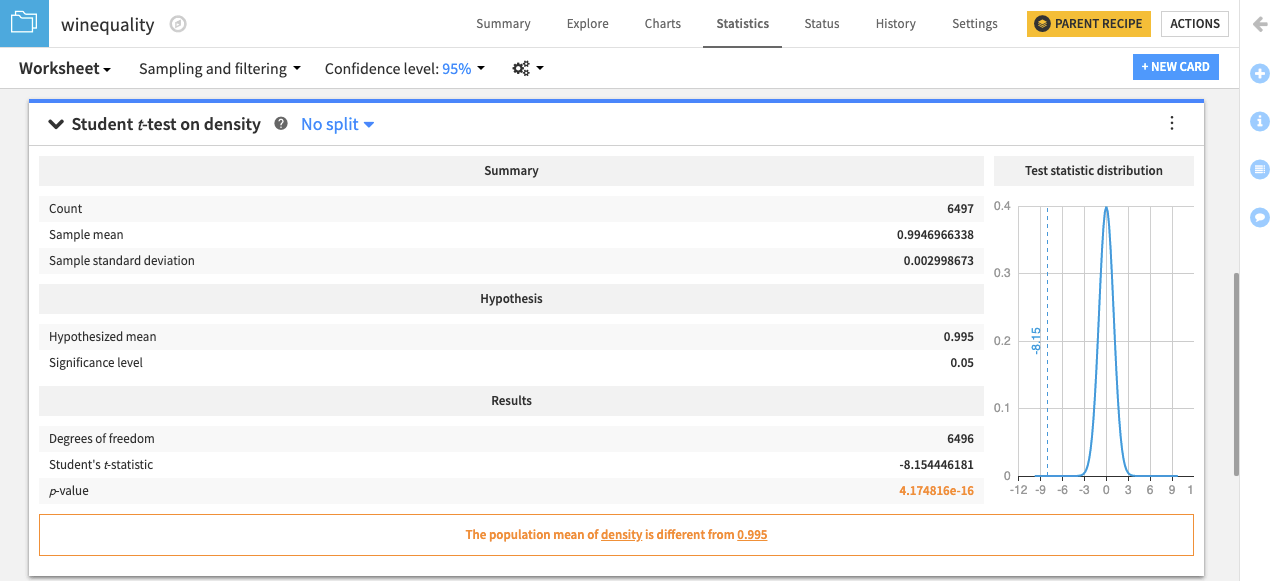Next, let’s go beyond describing the winequality dataset, by using inferential statistics to make quantitative decisions about the underlying population from which the dataset was drawn.

DSS enables you to perform hypothesis tests that include one-sample, two-sample, and N-sample tests on numerical variables, and to perform categorical tests on categorical variables.

# Hands-On: Perform One-sample Location Tests¶

Note

This lesson is a continuation of the Interactive Visual Statistics hands-on tutorial.

These tests allow you to compare the location parameters of a population to a hypothesized constant.

Let’s determine whether the mean of the underlying population for the density variable is equal to a specified value. To do this, we will use the one-sample Student t-test card.

• Click the New Card button from the “Worksheet” header, and then select Statistical tests. This brings up the “Statistical Tests” window.The left pane of the window lists four different categories for statistical tests (one-sample tests, two-sample tests, N-sample tests, and categorical tests). Clicking any of those categories shows the specific tests that are available within the category.

• Click One-sample test from the left column of the window, and then click Student t-test.

• Select density as the “Variable”, and specify 0.995 as the value for the “Hypothesized mean”.• Click Create Card to create the student t-test card on the density variable.The card displays a summary of the density variable, including its mean, the tested hypothesis, results of the test, and a plot of the distribution for the test statistic. The card also displays a conclusion about the test — in this case “The population mean of density is different from 0.995”. For more information about the student t-test card, see Student t-test (one-sample) in the reference documentation.

Similarly, you can test whether the median of the population for the density variable is equal to a specified value, using the Sign test (one-sample).